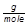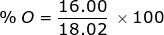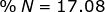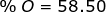Percent Composition Formula

Percent Composition Formula

Percent composition is the term used to describe the percent by mass of each element in a compound. It is typically found using the molar mass values for both the elements in the compound and the compound itself.Percent Composition Formula Questions:

1.Find the percent composition of water.

First, find the molar mass of water (H2O).

H = 1.01 x 2 = 2.02H2O = 2.02 + 16.00 = 18.02O = 16.00 x 1 =Now find the percent composition of each element.2. Find the percent composition of calcium nitrate, Ca(NO3)2.

Ca = 40.08 x 1 = 40.08Ca(NO3)2 = 40.08 + 28.02 + 96.00 = 164.10N = 14.01 x 2 = 28.02O = 16.00 x 6 = 96.00Related Links: Percent Composition Quiz